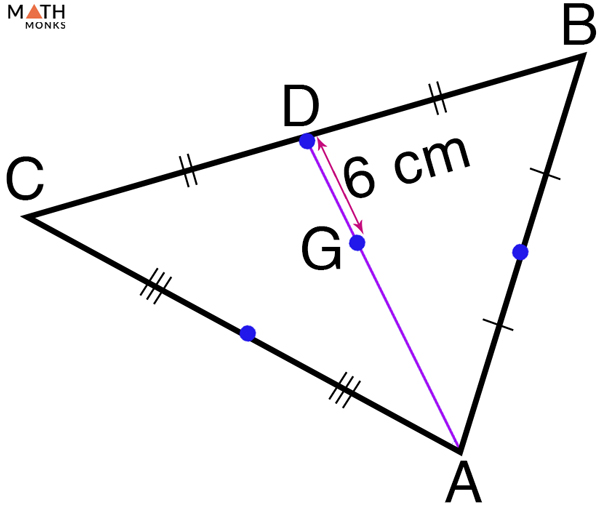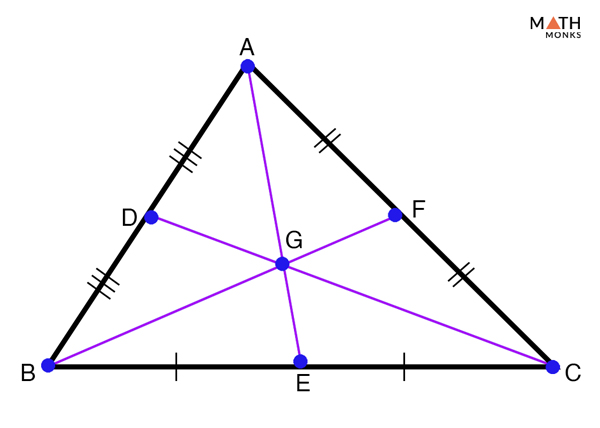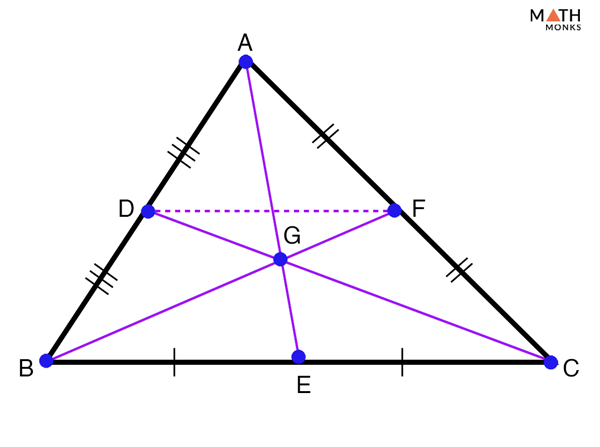# Centroid of a Triangle

The centroid is the geometric center of a two-dimensional plane figure. It is the arithmetic mean position of all the points in the figure. They are found in different geometric shapes. Here, in this article we will discuss about centroid in a triangle.

## What is Centroid of a Triangle

Every triangle has a single point within it that allows the triangle to balance perfectly, if the triangle is made of only one material. That point is called the centriod or the ‘center of gravity’ or ‘center of mass’ of the triangle.

Mathematically a centroid of a triangle is defined as the point where three medians of a triangle meet. It is one of the three points of concurrency in a triangle along with the incenter, circumcenter, and orthocenter. A centroid is represented typically by the symbol ‘G’.

Shown below is a ΔABC with centroid ‘G’.

## Properties

1. Found inside a triangle; in ΔABC, G is the centroid
2. Found at the intersection point of all three medians of a triangle and thus the point of concurrency of three medians.; AE, DC, & BF are the three medians that are concurrent and meet at G
3. The centroid divides each median into two segments, which are in the ratio of 2:1; here, centroid ‘G’ divides median AE into AG:GE, BF into BG:GF, and CD into CG:GD. All in the ratio of 2:1

## Formulas

### How to Find the Centroid of a Triangle Algebraically

To find the centroid of a triangle algebraically, we need to draw three medians one from each vertex of the triangle to the midpoint of their opposite sides.

Now, according to the 3rd property of centriod, it divides each median into two segments in the ratio of 2:1. Which means, centroid is 2/3 of the median’s distance from an interior angle and (1-2/3 = 1/3) 1/3 rd  of the median’s distance from the midpoint of the opposite side.

Given below is a triangle ΔABC, where three medians AE, BF, and CD meet at G to from the centriod of the triangle. Also note that E, F and D are the midpoints of sides BC, AC, and AB respectively.

Let us solve some examples to understand the concept better.

Given is a ΔABC where G is the centroid and AE, BF, & CD are the three medians. If the length of the median AE is 6 cm, what is the distance of the centroid from vertex A and the midpoint E?

Solution:

As we know,
AG = 2/3 AE, here AE = 6cm
= (2/3 × 6) = 4 cm
Similarly,
GE = 1/3 AE, here AE = 6cm
= (1/3 × 6) = 2 cmGiven that D is the midpoint of CB and G is the centroid of the triangle, if the length of GD is 6 cm, what is the length of AG?

Solution:

As we know, centroid divides each median into 2:1
Thus, we can write
AG/GD = 2/1, here GD = 6 cm
=> AG/6 = 2/1
=> AG = 6 × 2/1 = 12 cm

### How to Find the Centroid of a Triangle with Coordinates

If the coordinates of the three vertices of a triangle are (x1, y1), (x2, y2), (x3, y3), then the formula to find the centroid of the triangle is given below:

Let us solve some examples to understand the concept better.

Find the coordinates of the centroid of a triangle whose vertices are (5, 3), (1, -3) and (6, -3).

Solution:

The coordinates of the vertices are (5, 3), (1, -3) and (6, -3)
Here, the x-coordinates are:
x1 = 5, x2 = 1,x3 = 6
Similarly, the y-coordinates are:
y1 = 3, y2 = -3,y3 = -3
As we know,
The centroid of a triangle (G) = [(x1 + x2 + x3)/3, (y1 + y2 + y3)/3]
G = [(5 + 1 + 6)/3, (3 – 3 – 3)/3]
G = [(12/3), (-3/3)]
G = (4, -1)
Hence, the centroid of the triangle has coordinates (4, -1)

Triangle ABC has vertices A = (2, 4), B = (4, 12), and C = (6, 14). What are the coordinates of the centroid of triangle ABC?

Solution:

As we know,
The centroid of a triangle (G) = (x1 + x2 + x3/3, y1 + y2 + y3/3), here x1 = 2, x2 = 4,x3 = 6, y1 = 4, y2 = 12,y3 = -14
= (2 + 4 + 6/3, 4 + 12 + 14/3)
= (12/3, 30/3)
= (4, 10)

## Centroid Theorem of a Triangle

It is one of three properties of the centroid of a triangle discussed before.

### Centroid Theorem of a Triangle: ProofProve Centroid Theorem of a Triangle

To prove:

AG = 2/3 AE, BG = 23 BF, & CG = 2/3 CDProof:

Given: ΔABC,
AE, BF, & CD are the medians
E, F, & D are the midpoints of BC, AC, & AB respectively
G is the centroid
AC = 2AF
∠A =∠A (Common)
Thus,
DF ∥ BC and BC = 2DF
In ΔDGF and ΔCGB,
DF ∥ BC
Thus,
∠GDF = ∠GCB
∠EFG = ∠GBC
Thus,
ΔDGF ~ ΔCGB
As, BC = 2DF, the length of the sides of the triangles, ΔCGB and ΔDGF are in the ratio of 2:1
We have,
CG: GD = BG: GF = 2:1
Similarly, we can prove,
AG: GE = 2:1 and CG: GD = 2:1